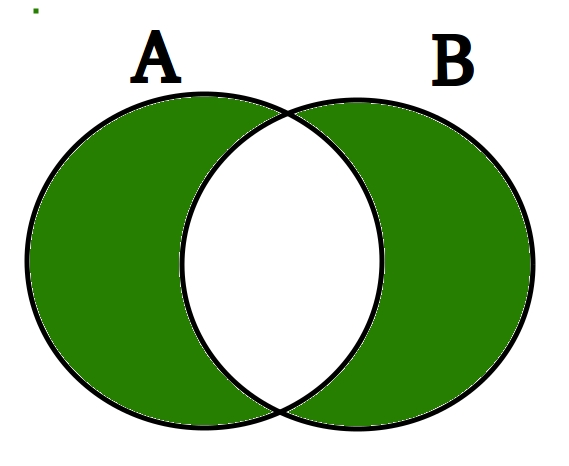# Python set symmetric_difference_update()

The symmetric difference of two sets is the set of elements which are in either of the sets but not in both of them.Symmetric Difference is marked in Green

symmetric_difference() method returns a new set which contains symmetric difference of two sets. The symmetric_difference_update() method updates the set calling symmetric_difference_update() with the symmetric difference of sets.

Syntax:
A.symmetric_difference_update(B)
Parameters:
The symmetric_difference takes a single “set” as a argument.
Returns:
This method returns None (which indicates absence of a return value). It only updates the set calling symmetric_difference_update() with the symmetric difference of sets.

CODE 1

 `# Python code to demonstrate working of  ` `# symmetric_difference_update() ` ` `  `A ``=` `{``'p'``, ``'a'``, ``'w'``, ``'a'``, ``'n'``} ` `B ``=` `{``'r'``, ``'a'``, ``'o'``, ``'n'``, ``'e'``} ` ` `  `# result is always none. ` `result ``=` `A.symmetric_difference_update(B) ` ` `  `print``(``'A = '``, A) ` `print``(``'B = '``, B) ` `print``(``'result = '``, result) `

Output:

```('A = ', set(['e', 'o', 'p', 'r', 'w']))
('B = ', set(['a', 'r', 'e', 'o', 'n']))
('result = ', None)
```

CODE 2

 `# Python code to demonstrate working of  ` `# symmetric_difference_update() ` ` `  `A ``=` `{``'s'``, ``'u'``, ``'n'``, ``'n'``, ``'y'``} ` `B ``=` `{``'b'``, ``'u'``, ``'n'``, ``'n'``, ``'y'``} ` ` `  `# result is always none. ` `result ``=` `A.symmetric_difference_update(B) ` ` `  `print``(``'A = '``, A) ` `print``(``'B = '``, B) ` `print``(``'result = '``, result) `

Output:

```('A = ', set(['s', 'b']))
('B = ', set(['y', 'b', 'u', 'n']))
('result = ', None)
```

My Personal Notes arrow_drop_upCheck out this Author's contributed articles.

If you like GeeksforGeeks and would like to contribute, you can also write an article using contribute.geeksforgeeks.org or mail your article to contribute@geeksforgeeks.org. See your article appearing on the GeeksforGeeks main page and help other Geeks.

Please Improve this article if you find anything incorrect by clicking on the "Improve Article" button below.

Article Tags :
Practice Tags :

1

Please write to us at contribute@geeksforgeeks.org to report any issue with the above content.